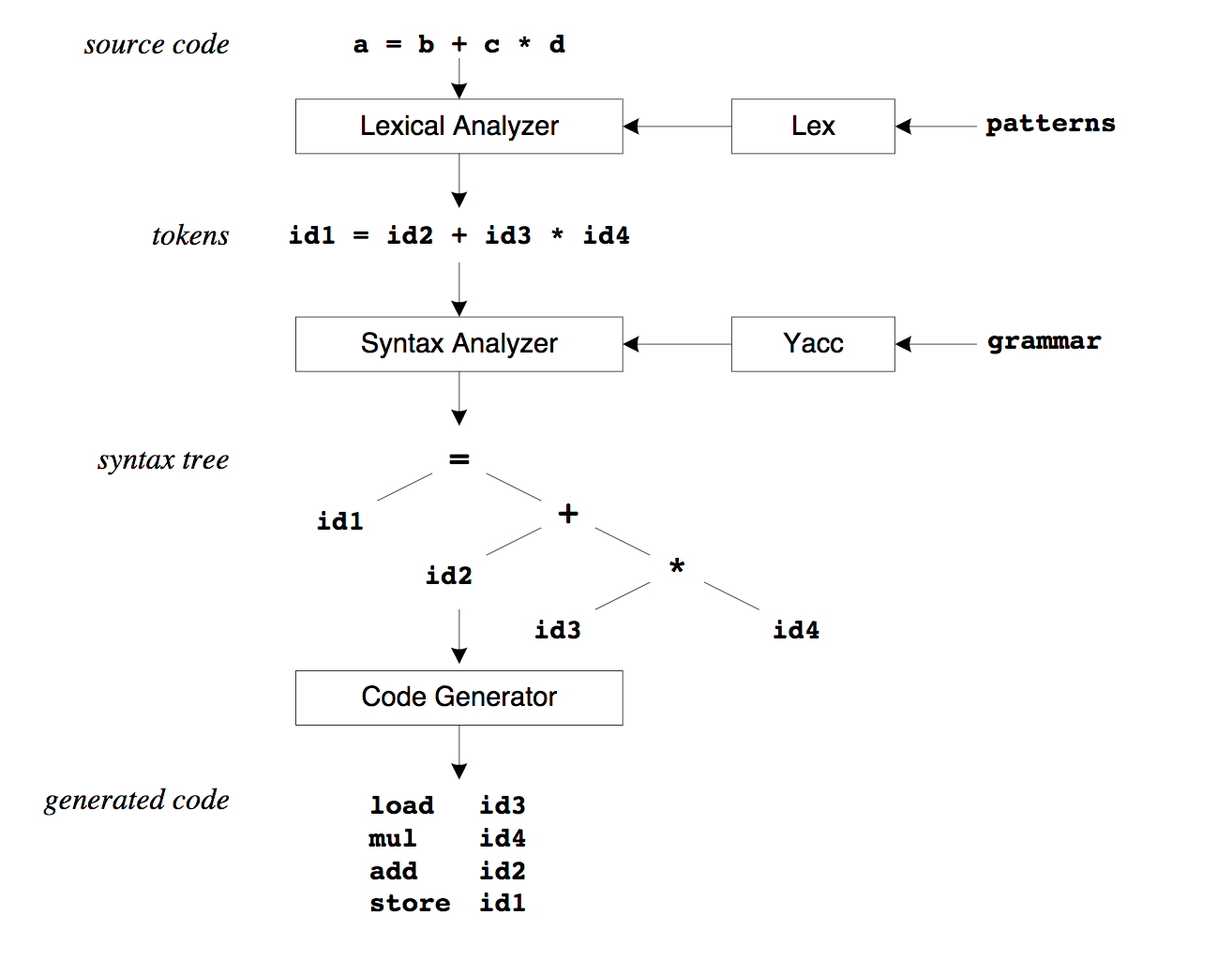More:

## Racc

2018-05-11

• Rex和Racc的关系
• 如何使用Racc进行自定义

### 1 TL;DR

Ruby中我们使用 sql-parser

```require 'sql-parser'
parser = SQLParser::Parser.new

# Build the AST from a SQL statement
ast = parser.scan_str('SELECT * FROM users WHERE id = 1')

# Find which columns where selected in the FROM clause
ast.select_list.to_sql
#=> "*"

# Output the table expression as SQL
ast.table_expression.to_sql
#=> "FROM users WHERE id = 1"

# Drill down into the WHERE clause, to examine every piece
ast.table_expression.where_clause.to_sql
#=> "WHERE id = 1"
ast.table_expression.where_clause.search_condition.to_sql
#=> "id = 1"
ast.table_expression.where_clause.search_condition.left.to_sql
#=> "id"

```

1. patterns, 用 `parser.rex` 来配置, 如 SELECT, ORDER 等词汇
2. grammar, 用 `parser.racc` 来配置, 定义了语法, 即 SELECT, ORDER 的组合方式

```SELECT * FROM orders WHERE id = 1

```

```SELECT
*
FROM
WHERE

```

### 2 Rex 和 Racc 的来源

#### 2.1 Yacc和Lexical

Racc和Rex分别来源于单词 Yacc`Lexical Analyser`

1. 有哪些词汇(patterns)?
2. 有哪些语法(grammar)?### 3 Rex

#### 3.1 示例

```macro
DIGIT_MACRO     \d+

rule
{DIGIT_MACRO} { [:DIGIT, text.to_i] }

```

```输入 1024, 解析为词汇 [:DIGIT, 1024]

```

### 4 Racc

#### 4.1 示例

```rule
expression
:
DIGIT
| DIGIT ADD DIGIT { return val + val }
| DIGIT SUBSTRACT DIGIT { return val - val }
| DIGIT MULTIPLY DIGIT { return val * val }
| DIGIT DIVIDE DIGIT { return val / val }
end

```

1. expression 仅仅是一个名词, 可以起名为abc, bcd都行, 他可以被复用, 即规则可以嵌套使用. ":"后面是代表各种不同的情况, | 代表 或
2. DIGIT ADD SUBSTRACT 等, 均为 calculator.rex 中申明的词汇(patterns)
3. val是指匹配成功之后, 对应的值

如字符串 `2 + 2`, 匹配到了 `DIGIT ADD DIGIT` 这部分, 则

```val = 2
val = "+"
val = 2

```
4. {} 表示的是ruby代码, 即如何处理该语法.

### 5 编译

```rex calculator.rex -o calculator.rex.rb
racc calculator.y -o calculator.racc.rb

```

```pry -r ./calculator.racc.rb

```

```Calculator.new.parse("2 + 2") #=> 输出 4
Calculator.new.parse("2 - 2") #=> 输出 0
Calculator.new.parse("2 * 3") #=> 输出 6
Calculator.new.parse("2 / 1") #=> 输出 2
Calculator.new.parse("2 | 1") #=> 抛出异常, 因为 | 无法解析: parse error on value 2 (DIGIT)

```